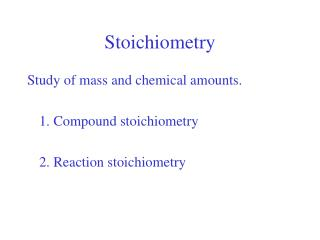DownloadDownload PresentationStoichiometry

# Stoichiometry

Télécharger la présentation## Stoichiometry

- - - - - - - - - - - - - - - - - - - - - - - - - - - E N D - - - - - - - - - - - - - - - - - - - - - - - - - - -
##### Presentation Transcript

1. Stoichiometry Study of mass and chemical amounts. 1. Compound stoichiometry 2. Reaction stoichiometry

2. Connecting the Macro and Atomic Scales: The Mole Chemical macroscopic counting unit: 1 mole contains 6.022 x 1023 particles 6.022 x 1023 is Avogadro’s number, named after Amedeo Avogadro. Avogadro’s number is not special, like pi, but is invented by us for our convenience.

3. Why it works. • The mass of 1 mol of an element is equal to its atomic mass in grams • Molar mass is in g/mol • Molar mass of Al = 26.98 g/mol • Unit sign: mole = mol sometimes, molar mass = M

4. How it works Two new conversion factors: Avogadro’s number: Molar mass:

5. How many atoms are in 2.60 mol of Ne?

6. How many atoms are in 3.84 mol of B? • 3.84 • 2.31 x 1025 • 2.31 x 1024 • 6.38 x 10-24

7. How many moles do 4.00 x 1022 atoms of Ne make?

8. How many moles do 6.84 x 1021 atoms of B make? • 6.84 x 1021 mol • 0.0114 mol • 4.12 x 1045 mol • 6.84 x 10-21 mol

9. How many moles do 45.0 g of Ne make?

10. How many moles do 45.0 g of Al make? • 1.67 mol • 1214 mol • 7.47 x 10-23 mol • 2.71 x 1025 mol

11. What is the mass of 2.60 mol of C?

12. What is the mass of 2.60 mol of O? • 8.90 g • 0.163 g • 2.60 g • 41.6 g

13. How many moles are represented by 1.00 g of C?

14. How many atoms are in 1.00 g of O?

15. What is the mass of one Au atom?

16. How many C atoms are in a 1.00 caret diamond?

17. Compounds and Moles • 1 mole of a compound contains 6.022 x 1023 molecules or “units” • Molar mass = sum of atomic molar masses • H2O • CO2 • Fe(NO3)3

18. Molecules, Atoms and Moles Consider UF6 • 0.50 mol UF6 contains …

19. Language for nonmolecular compounds … 1 mol CO2 contains 6.022 x 1023molecules 1 mol NaCl contains 6.022 x 1023formula units

20. Percent Composition What fraction of a compound is made up of one of its elements? Consider FeO …

21. Determining Formulas from Percent Composition The key: the ratio of atoms in the formula is the same as the ratio of moles of those elements

22. Determining Formulas from Percent Composition The key: the ratio of atoms in the formula is the same as the ratio of moles of those elements If you determine the ratio of moles, you know the formula.

23. Determining Formulas from Percent Composition The key: the ratio of atoms in the formula is the same as the ratio of moles of those elements If you determine the ratio of moles, you know the formula. But! You get the empirical formula.

24. Example: A compound has Ca, S, and O. Ca: 29.45% S: 23.55% O: 47.00% What is the empirical formula?

25. Empirical vs. Molecular Formulas Example: Ethene is C2H4 Percent composition tells us mol H/mol C = 2 The empirical formula is CH2. The molecular formula is C2H4.

26. Example: A hydrocarbon has 82.65% C and 17.34% H Molar mass is 58.12 g/mol What are the empirical and molecular formulas?

27. Hydrated Compounds Solids in which molecules of water are trapped and become part of the compound. Ex: Gypsum: CaSO4 • 2H2O is hydrated calcium sulfate CaSO4 is anhydrous calcium sulfate

28. Determining the number of waters of hydration 1.023 g CuSO4• x H2O is heated to drive off the water. The resulting anhydrous CuSO4 has a mass of 0.654 g. What is the value of x?